# 7 1 Describing Reactions Chapter 7 Chemical Reactions

• Slides: 15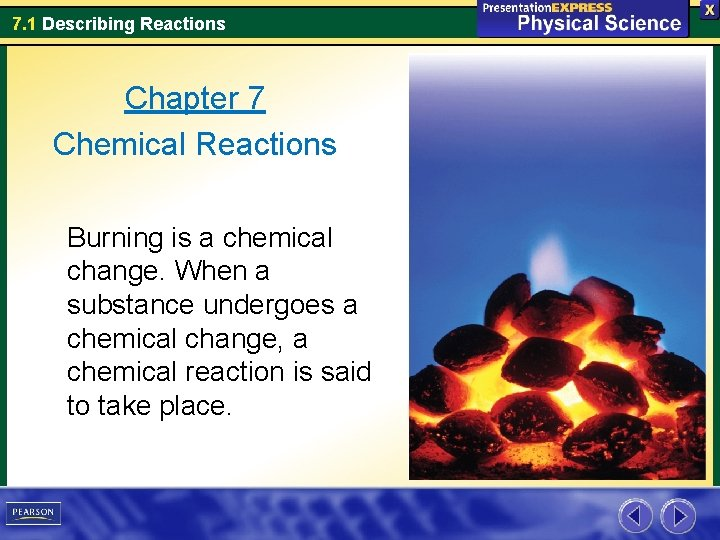7. 1 Describing Reactions Chapter 7 Chemical Reactions Burning is a chemical change. When a substance undergoes a chemical change, a chemical reaction is said to take place.7. 1 Describing Reactions 7. 1 Key Concepts What is the law of conservation of mass? Why must chemical equations be balanced? Why do chemists use the mole? How can you calculate the mass of a reactant or product in a chemical reaction?7. 1 Describing Reactions Chemical Equations A useful description of a chemical reaction tells you the substances present before and after the reaction. • The substances that undergo change are called reactants _________. • The new substances formed as a result of that products change are called ________. Reactants Products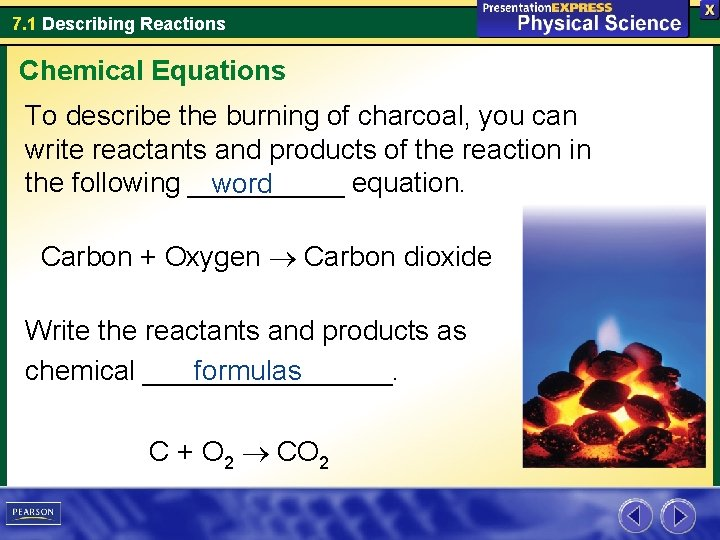7. 1 Describing Reactions Chemical Equations To describe the burning of charcoal, you can write reactants and products of the reaction in the following _____ equation. word Carbon + Oxygen Carbon dioxide Write the reactants and products as formulas chemical ________. C + O 2 CO 27. 1 Describing Reactions Chemical Equations A chemical equation is a representation of a chemical reaction in which the reactants and products are expressed as formulas. You can read the equation C + O 2 CO 2 as react • Carbon and oxygen _______ and form carbon dioxide ____ • The reaction of carbon and oxygen _______ carbon dioxide. ” yields7. 1 Describing Reactions Chemical Equations Conservation of Mass During chemical reactions, the mass of the equal products is always _______ to the mass of the reactants. – This principle is the law of conservation of mass. When charcoal burns, the mass of the carbon dioxide produced is equal to the mass of the charcoal and oxygen that reacted. C + O 2 CO 27. 1 Describing Reactions Chemical Equations Whether you burn one carbon atom or six carbon atoms, the equation used to describe the reaction is always the same.7. 1 Describing Reactions Balancing Equations In order to show that mass is conserved during a reaction, a chemical equation must be balanced _________. You can balance a chemical equation by changing the _________, the numbers that appear coefficients before ______ the formulas.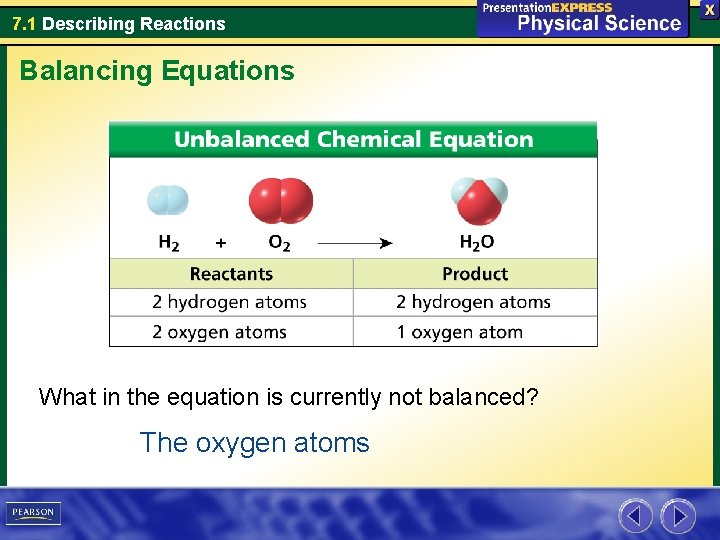7. 1 Describing Reactions Balancing Equations What in the equation is currently not balanced? The oxygen atoms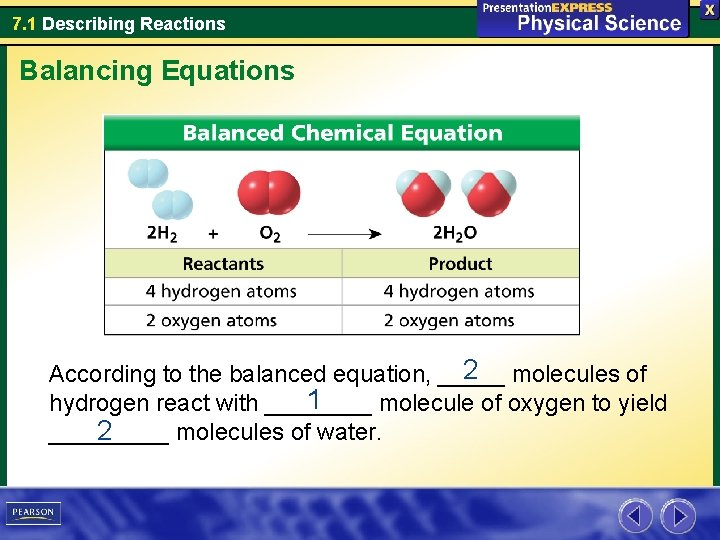7. 1 Describing Reactions Balancing Equations 2 molecules of According to the balanced equation, _____ 1 hydrogen react with ____ molecule of oxygen to yield 2 _____ molecules of water.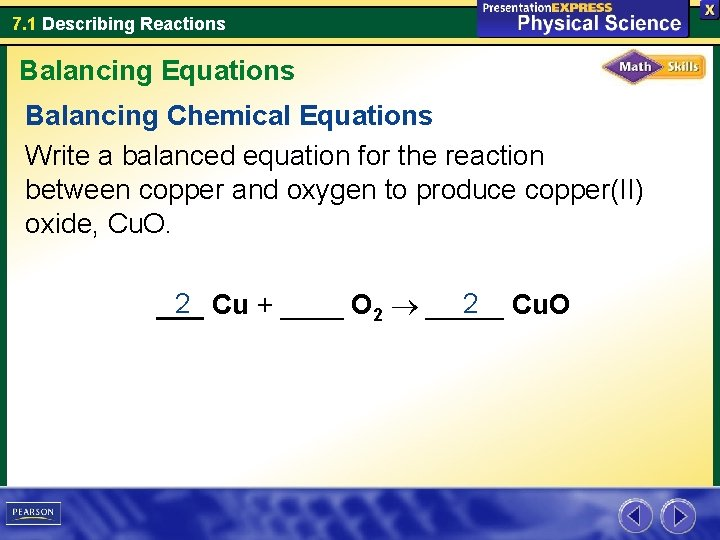7. 1 Describing Reactions Balancing Equations Balancing Chemical Equations Write a balanced equation for the reaction between copper and oxygen to produce copper(II) oxide, Cu. O. 2 Cu + ____ O 2 _____ 2 Cu. O ___7. 1 Describing Reactions Describing Ionic Compounds Balance the following chemical equations. 2 H 2 O 2 ____ 2 H 2 O + ____ O 2 a. ____ 2 HCl ____ H 2 + _____ Mg. Cl 2 b. ____ Mg + ____7. 1 Describing Reactions Assessment Question 1 Which substance below is a product? carbon dioxide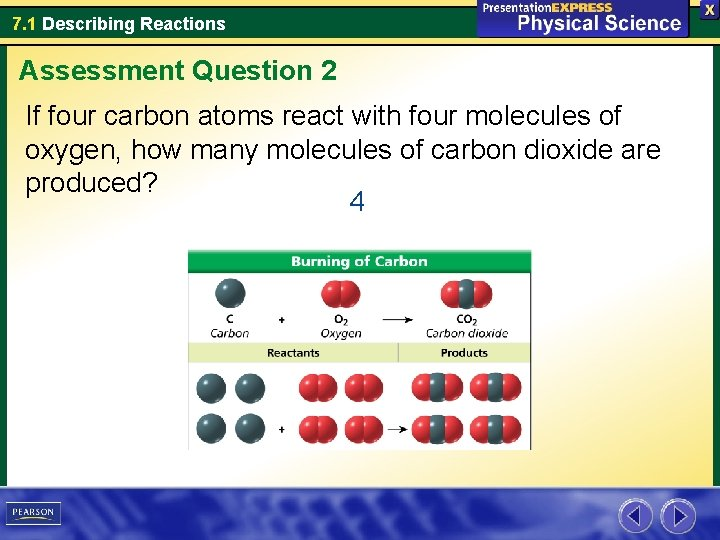7. 1 Describing Reactions Assessment Question 2 If four carbon atoms react with four molecules of oxygen, how many molecules of carbon dioxide are produced? 4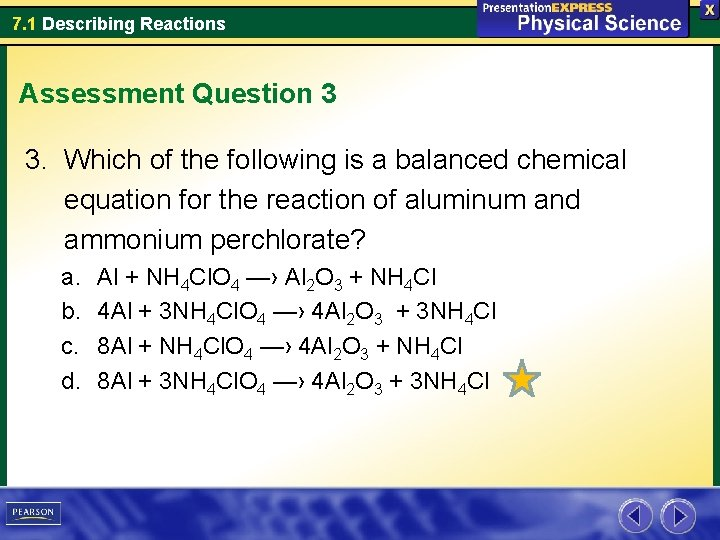7. 1 Describing Reactions Assessment Question 3 3. Which of the following is a balanced chemical equation for the reaction of aluminum and ammonium perchlorate? a. b. c. d. Al + NH 4 Cl. O 4 —› Al 2 O 3 + NH 4 Cl 4 Al + 3 NH 4 Cl. O 4 —› 4 Al 2 O 3 + 3 NH 4 Cl 8 Al + NH 4 Cl. O 4 —› 4 Al 2 O 3 + NH 4 Cl 8 Al + 3 NH 4 Cl. O 4 —› 4 Al 2 O 3 + 3 NH 4 Cl